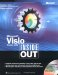# Exploring Shape Geometry

3 4

If you want to design your own shapes with the idea of adding SmartShapes formulas, you need to understand specifically how the drawing tools operate on shape geometry. When you strip away the formulas and formatting, shapes are just geometry—that is, lines, and arcs—and that's how Visio represents them internally. Every time you draw a new shape, Visio records your actions in a ShapeSheet spreadsheet for the shape. Every shape has a Geometry section in its ShapeSheet that defines each point that makes up the shape, as Figure 25-6 shows.

Depending on the tool used to create a shape, the Geometry section can describe points on a line, arc, ellipse, or spline. You can even convert one type of segment to another with the Change Row Type command on the Edit menu. Much of what the ShapeSheet records about shape size and position is also displayed in the Size & Position window and on the status bar at the bottom of the Visio window.

Visio defines shape geometry dynamically in terms of a shape's width and height by using default formulas. The first point on a shape—that is, the first point created by a drawing tool—is described in the MoveTo cell of the Geometry 1 section. The formula in the X column of this cell frequently looks like this:

` =Width*0 `Figure 25-6.  Every shape is described in the Geometry section of its ShapeSheet.

This formula multiplies the value of the Width cell by zero to describe the horizontal starting point of the first line segment, which is the far left side of the shape. A similar formula in the Y column of the MoveTo cell multiplies the value of the Height cell by zero, which is the bottom of the shape. Therefore, the shape's origin, or 0,0 point in coordinate terms, is the bottom, left corner. If you click either MoveTo cell while the drawing page is visible, you'll see that Visio confirms this coordinate by selecting the lower left vertex, as Figure 25-7 shows. The subsequent rows in the section reveal the type of segment: line, arc, circular arc, elliptical arc, and so on.Figure 25-7.  In the Geometry section, selecting a cell also selects the corresponding vertex on the shape.Microsoft Visio Version 2002 Inside Out (Inside Out (Microsoft))
ISBN: 0735612854
EAN: 2147483647
Year: 2000
Pages: 211
Authors: Nanette Eaton